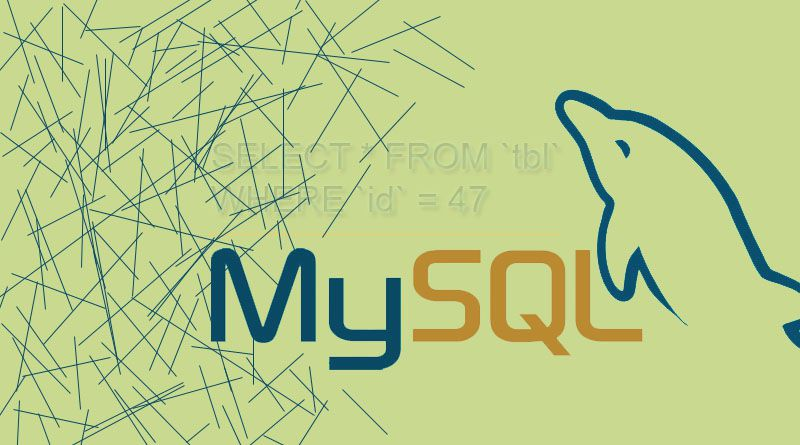# Referential integrityIt is relational database concept in which multiple tables shares consistent relationship based on the data stored on tables.

For example, a school X has 2 tables, a student table, and a student fee table. In the student table we have 2 columns – the student ID and the student name. In the student fee table, we have 2 columns – the student ID and the fee for the given ID.

Now, suppose we want to remove a student because he no longer study at school X. So we need to remove that student from student table. As the student ID from student table exists in student fee table then from that table we need to delete too manually. That’s a big deal if there are too many table attached.

By referential integrity we can solve this problem. Lets define the student ID column in the student table to be our primary key. Then define the student ID column in the student fee table to be a foreign key that points to a primary key that is the student ID column in the student table.

Once we define our foreign to primary key relationship, we need to add ‘constraint’ on student fee table i.e. ‘cascading delete’. Whenever an student is removed from the student table, any data that student has in the student fee table would automatically be removed from the student fee table.

#### Referential integrity example:

ALTER TABLE `tbl_1` ADD CONSTRAINT `tbl_std_constr` FOREIGN KEY (`student_id`) REFERENCES `tbl_2` (`student_id`) ON DELETE CASCADE ON UPDATE CASCADE;